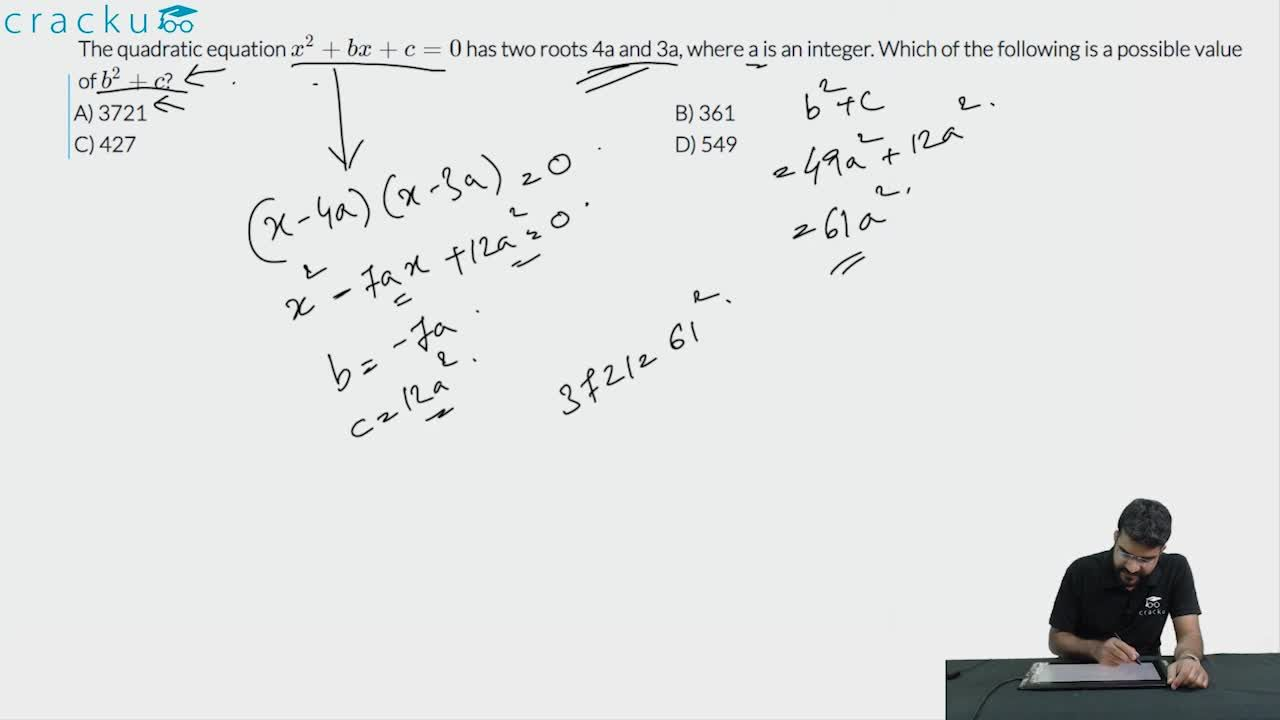Question 75

# The quadratic equation $$x^2 + bx + c = 0$$ has two roots 4a and 3a, where a is an integer. Which of the following is a possible value of $$b^2 + c$$?

Solution

Given,

The quadratic equation $$x^2 + bx + c = 0$$ has two roots 4a and 3a

7a=-b

12$$a^2$$ = c

We have to find the value of  $$b^2 + c$$ = 49$$a^2$$+ 12$$a^2$$=61$$a^2$$

Now lets verify the options

61$$a^2$$ = 3721 ==> a= 7.8 which is not an integer

61$$a^2$$ = 361 ==> a= 2.42 which is not an integer

61$$a^2$$ = 427 ==> a= 2.64 which is not an integer

61$$a^2$$ = 549 ==> a= 3 which is an integer

### View Video Solution